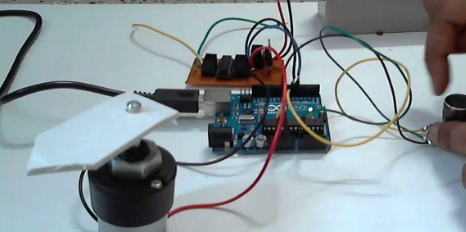In this project, we have described how the speed can be handled by rotating the knob of the potentiometer.

Summery

In this project, we have described how the speed can be handled by rotating the knob of the potentiometer.DC motor is the usually utilized motor in Robotics and in various electronics projects. For handling the speed of DC motor we have several methods, like with relays, etc. In this circuit, for handling the speed of the DC motor, we utilize a 100K ohm potentiometer to modify the duty cycle of the PWM signal.

For Instance, if we sustain 256 values to the analog input, then the HIGH time will be (1024-256)ms and LOW time will be 256ms. Hence, it means the duty cycle is 75%. So that’s how we can execute the DC Motor Speed Control using Arduino and potentiometer.

int pwmPin = 12; // assigns pin 12 to variable pwm
int pot = A0; // assigns analog input A0 to variable pot
int c1 = 0;   // declares variable c1
int c2 = 0;   // declares variable c2

void setup()  // setup loop
{
pinMode(pwmPin, OUTPUT);
pinMode(pot, INPUT);
}

void loop()
{